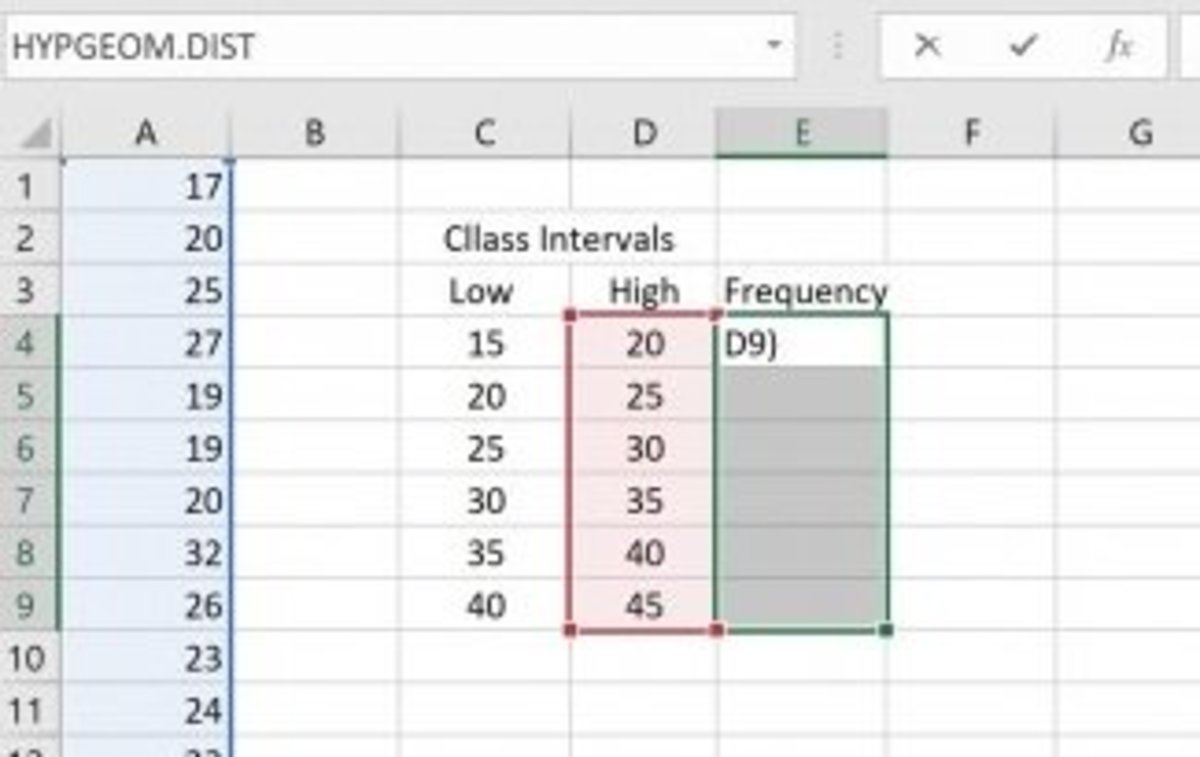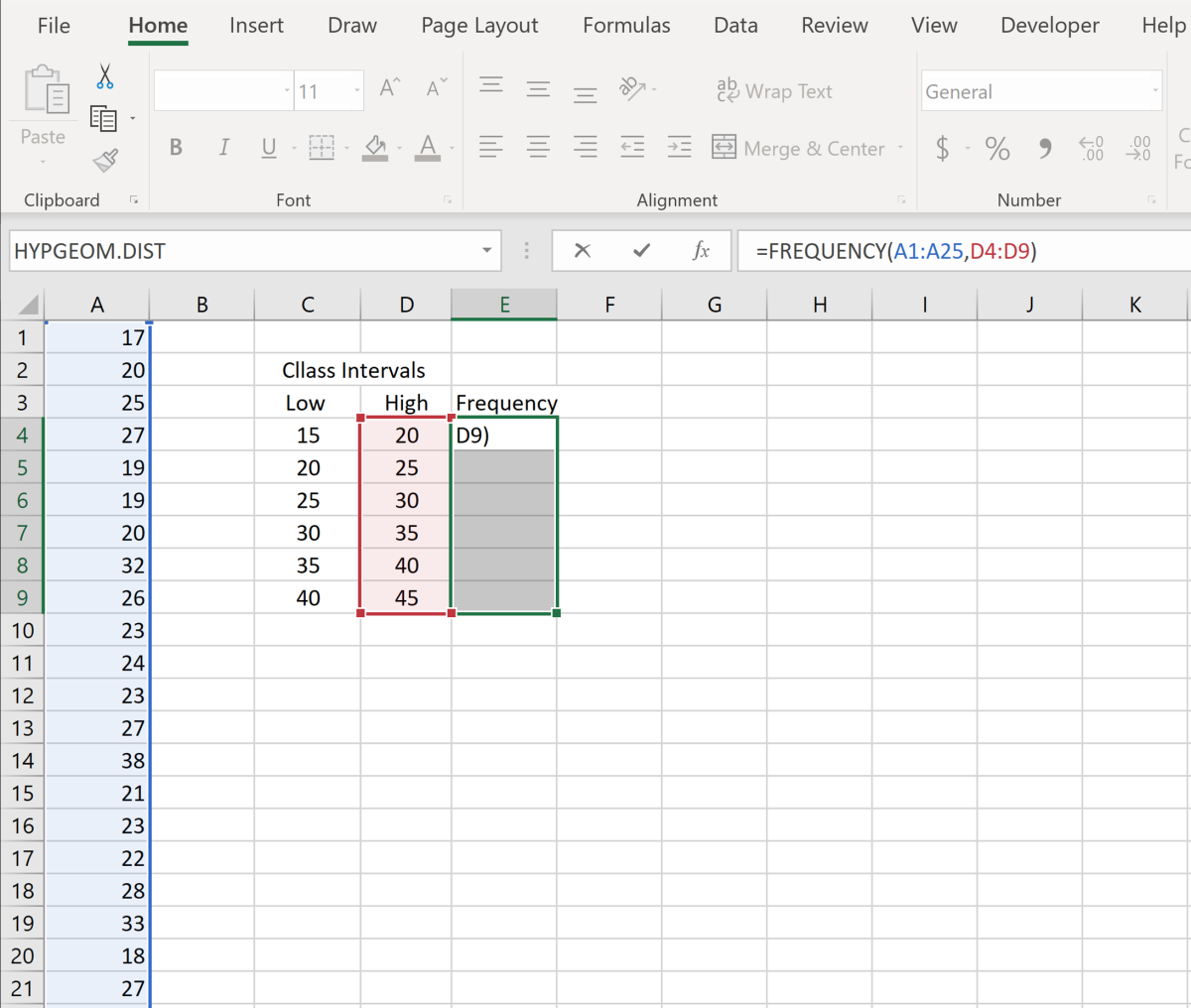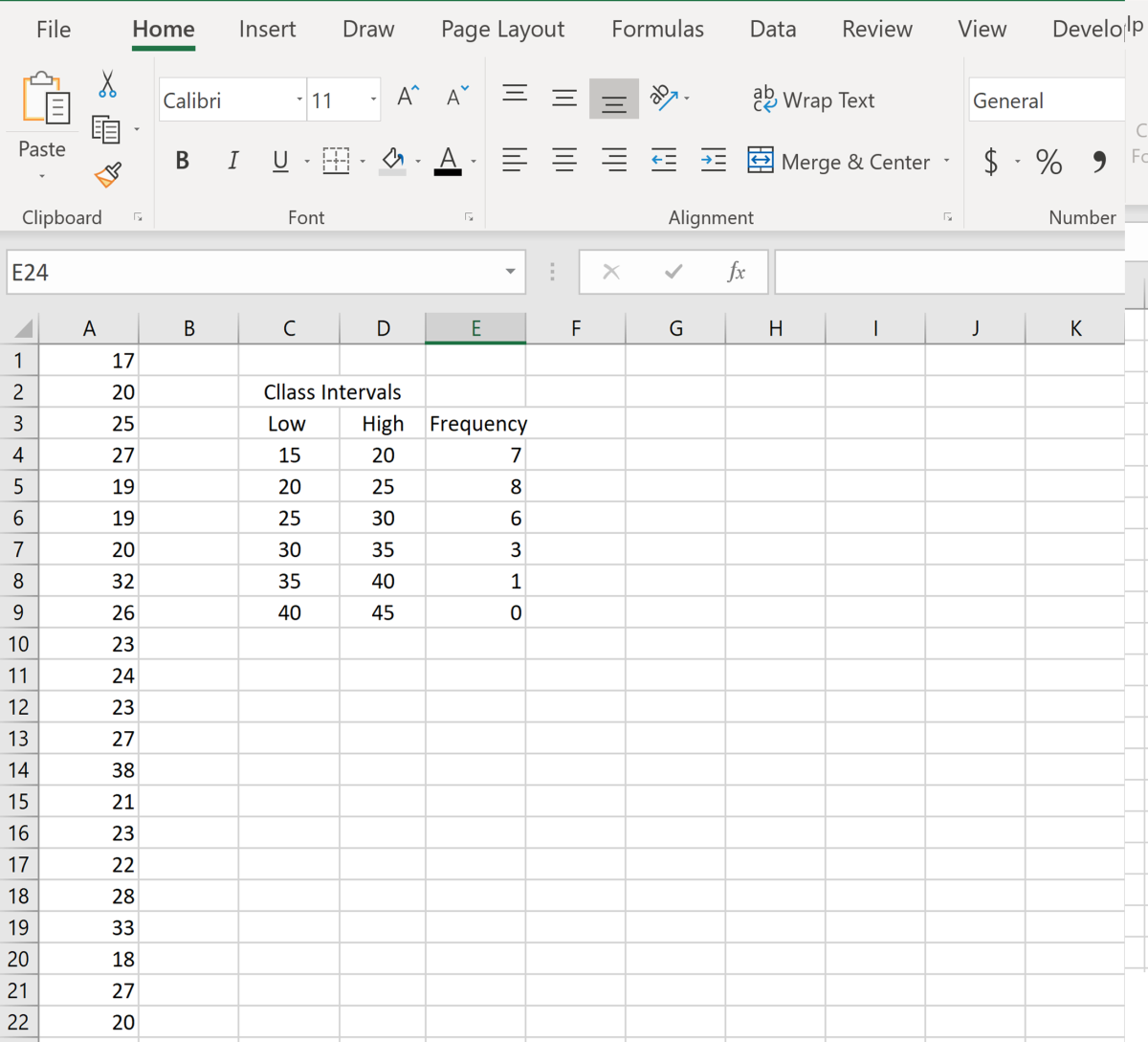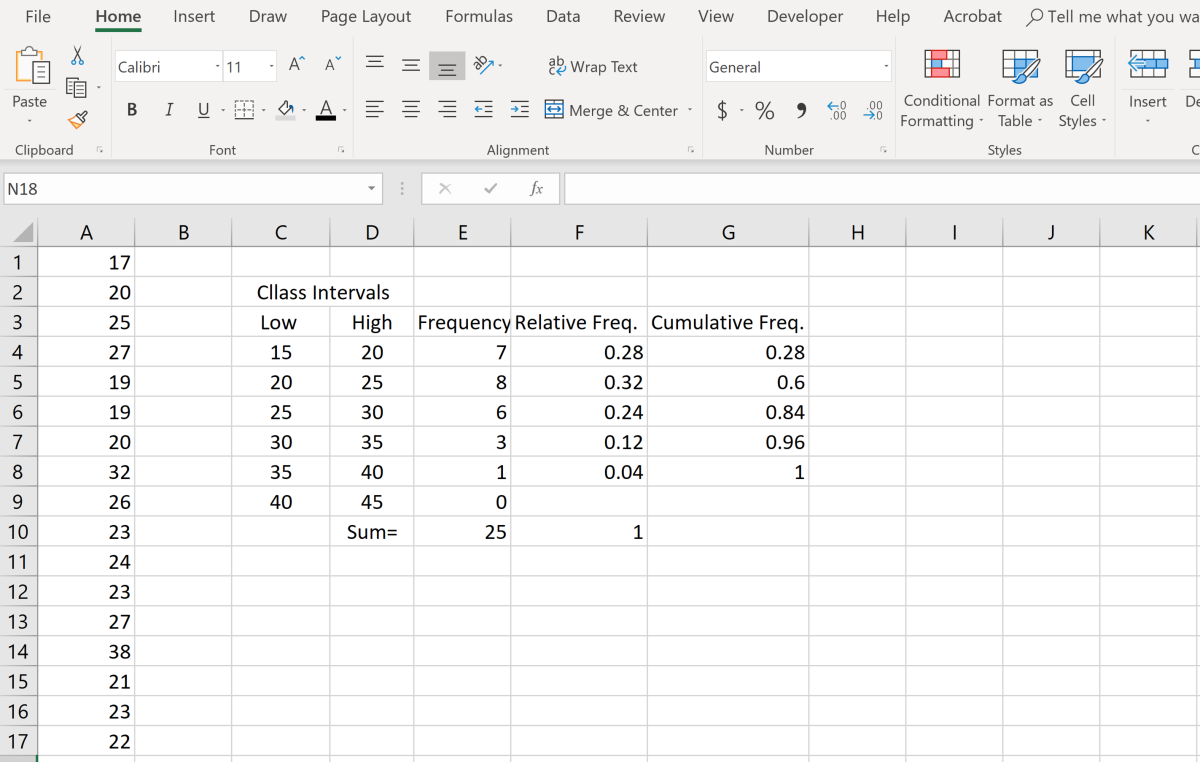# How to Create a Frequency Distribution Table in Excel

Joshua is a graduate student at the USF. He has interests in business technology, analytics, finance, and lean six sigma.The illustration shows a frequency distribution that was created in an Excel worksheet. Created by Joshua Crowder

## What Is a Frequency Distribution?

In the field of statistics, a frequency distribution is a data set or graph that is organized to show frequencies of the occurrence outcomes. Each outcome from the data set that forms a frequency distribution is a repeatable event and can be observed multiple times.

A simple example is the frequency of book purchases. Since the shelves of a book stores have multiple books for sale, the frequency of purchases as an outcome is repeatable. Frequency distributions can be shown in the form of a table and in graph form. Frequency distributions have proved to be most useful particularly in summarizing large data sets and assigning probabilities to attributes of that data.

## Excel Frequency Function

The Excel frequency function calculates the frequency of values that occur within a range of values. So, if one of the ranges in a table is 15–20 and only 2 numbers in the data set are between this range, then the frequency will show up as 2. Since this function returns an array (data set), as a result, the formula must have a corresponding array for an input. The Syntax of the frequency function is shown in the table below.

## Characteristics of Frequency Function

=FREQUENCY(data_array, bins_array)

data_arrry: reference to the data set that is counted

bins_array: reference to intervals to group the data

## Frequency Function Example

It would be best to go right into an example, as this function can cause some confusion in a general explanation. Suppose you have a list of numbers, and you need to know the frequency of the numbers in multiple ranges or class intervals. Consider the following data set: 17, 20, 25, 27, 19, 19, 20, 32, 26, 23, 24, 23, 27, 38, 21, 23, 22, 28, 33, 18, 27, 20, 23, 27, 31.

Before creating a table, you need to decide how many classes of intervals you will have in the frequency table that you are creating. The best way to find this out is by taking the square root of the numbers that you have in the data set. Since I have 25 numbers in this set, it would be suitable to separate the data into 5 classes.

Next, to get an idea of where the minimum class should start, you need to look at the minimum value in the data set. Since the minimum is 17, I'll choose 15 to be the minimum of the first class interval. Now I can create all the class intervals based on this first minimum value. Observe the table below. While also considering my maximum value from the data set, I created five classes. It worked out this time, but if the high value was 41, I would have had to create another class interval.

## Creating the Class Interval

Lower Class Upper Class

15

20

20

25

25

30

30

35

35

40

## Applying the Frequency Function

Now that the class intervals are created the frequency function can be applied. The area to the right of my class intervals is where the frequencies will appear. This whole area needs to be selected.

Next, the formula needs to be typed in. It will appear as =frequency(, then after the first parenthesis the data set needs to be selected. After the data set is selected a comma is added, then the array of high-class values is selected. Next, add a parenthesis at the end and pause.

To enable the function, you need to press ctrl + shift + enter. After doing so, all the frequencies will populate. The control shift enter task adds brackets to the ends of the function. Note that the function will not work properly without this last step.

When using this function, you have to be very specific about the intervals. For instance, if I had a value below 15 it would still show up in my first-class interval because the function only knows that everything under the value of 20 goes in the lowest class interval. Same with the highest class. If I had numbers above 45, they would still be counted as being in the highest class.

Scroll to ContinueThis is the point where you press shift+ctrl+enter. Doing so will add brackets to the function to enable it to print all the frequency values. Created by Joshua Crowder

## Results

Below, the illustration shows the results of the frequency function. Notice that the sum of the frequencies adds up to a total of 25, which is the total number of values in the example data set. Take notice that I added an extra class by accident and that the frequency for that class remains at zero.

The way I used this formula every value less than or equal to 20 appears in the first class. In the next class, all numbers are greater than 19 and less than or equal to 25. If you need to exclude the higher interval value, label them as 19.99, 24.99, 29.99, 34.99, 39.99 when using this function.The frequencies in this case are the number of individual numbers in the data set that appears within each interval. Created by Joshua Crowder

## Adding Relative Frequency and Cumulative Frequencies

Depending on how your data set needs to be analyzed, adding relative frequency and cumulative frequency columns to a frequency table can be beneficial. As shown in the illustration below, I created an additional column to show the relative frequency.

First, for the relative frequency column, I summed the frequencies, then divided each frequency by the sum to show each proportion. Next, for the cumulative frequency column, I added the cumulative frequency for each consecutive class.It is crucial that the sum of the relative frequencies is 1 and that the cumulative frequency for the last class should be 1. If either is not 1, an error in the calculation may have been made. Created by Joshua Crowder

To learn more about using creating frequency distributions and other applications related to Excel statistical applications I recommend the following book. I have been using the Excel Bible for years to improve my understanding of all aspects of this Microsoft product.

Britannica, T. E. (2013, December 10). Frequency distribution. Retrieved October 16, 2018, from https://www.britannica.com/technology/frequency-distribution

FREQUENCY function. (n.d.). Retrieved October 16, 2018, from https://support.office.com/en-us/article/FREQUENCY-function-44E3BE2B-ECA0-42CD-A3F7-FD9EA898FDB9

Crowder, J. (2020, January 1). How to Create a Frequency Distribution Table in Microsoft Excel. Retrieved January 1, 2020, from https://youtu.be/TNBsbEL_UGY.

This content is accurate and true to the best of the author’s knowledge and is not meant to substitute for formal and individualized advice from a qualified professional.